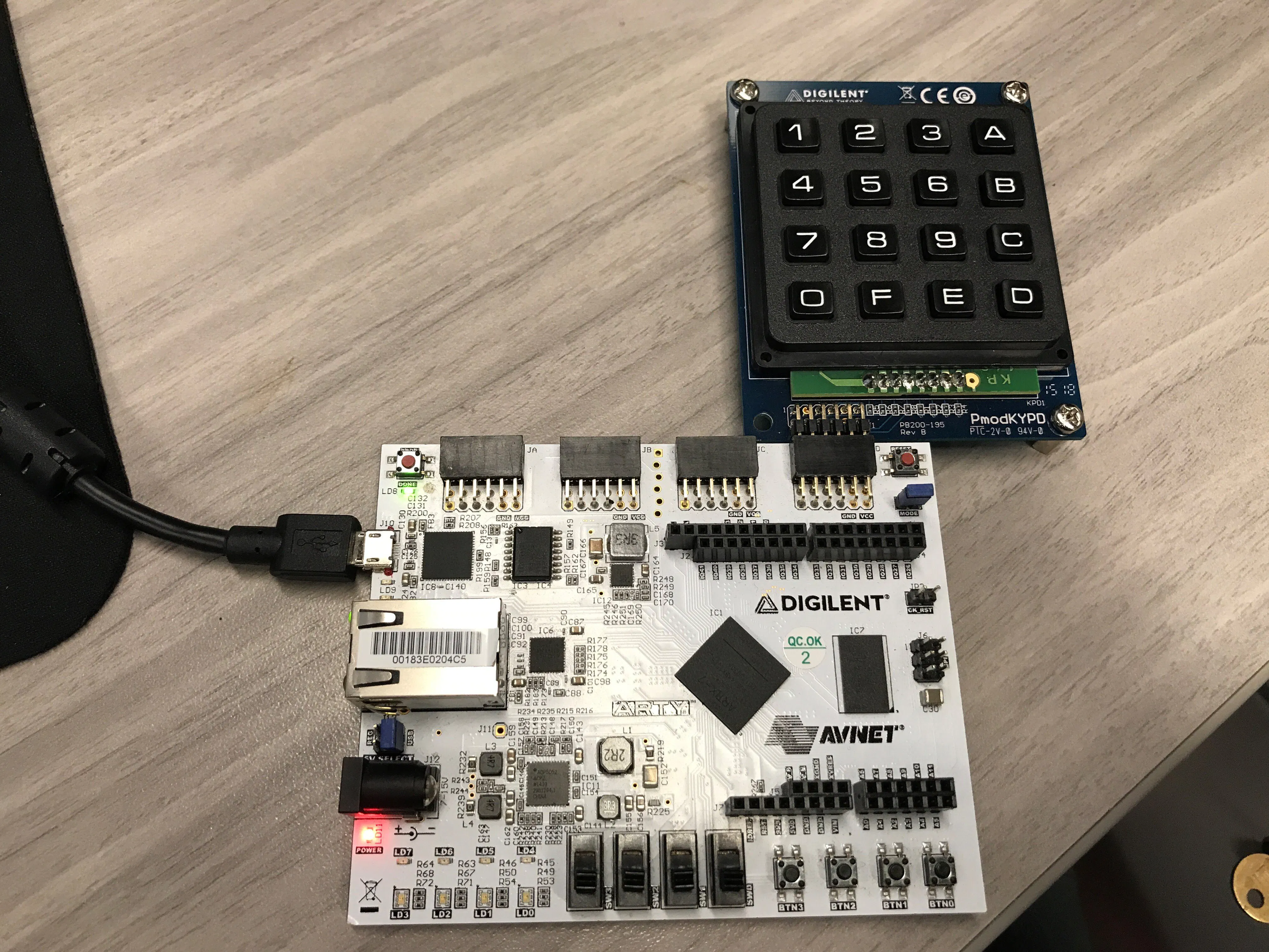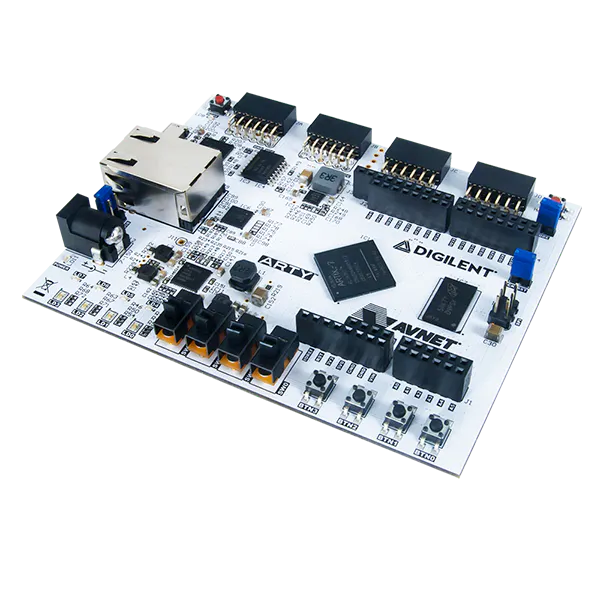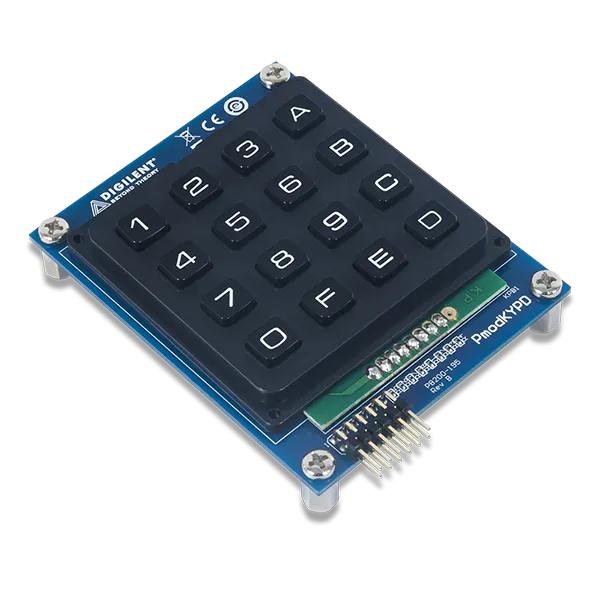Published

# Arty Dozenal Calculator

A simple calculator that operates on dozenal (base-12) numbers.

BeginnerShowcase (no instructions)1 hour1,501## Things used in this project

### Hardware componentsDigilent Arty A7-35T
×1Digilent Pmod KYPD
×1

### Software apps and online services

 Tera Term

## Code

### main.c

C/C++
See the "Setting up the Project" section above for instructions on using this file.
```#include "PmodKYPD.h"
#include "sleep.h"
#include "xil_cache.h"
#include "xil_io.h"
#include "xparameters.h"

#define KYPD_KEYTABLE "0*/-789+456E123X"

#define MAX_LENGTH 32

char parseInput(char *input, int *operand1, int *operand2);
int  dozToDec(char digit);
int  decToDoz(char *result, int num);
int  isOperator(char ch);
int  evaluate(int op1, int op2, char operator);
void enableCaches();
void disableCaches();

int main() {
enableCaches();

print("Begin\n\n\r");

u16 keystate;
XStatus status, lastStatus = KYPD_NO_KEY;
u8 key, lastKey = 'z';

char input[MAX_LENGTH];
while (1) {
int i = 0;
while (btn == 0) {
if (status == KYPD_SINGLE_KEY
&& (status != lastStatus || key != lastKey)) {
input[i] = key;
xil_printf("%c", key);
lastKey = key;
i++;
}
lastStatus = status;
usleep(1000);
}
for(; i < MAX_LENGTH; i++) {
input[i] = '\0';
}
if (btn == 8) {
break;
}
while (btn) {
btn = READ_BTN; // Wait for button to be released
}
int op1, op2;
char operator = parseInput(input, &op1, &op2);
int resultDec = evaluate(op1, op2, operator);
char resultDoz[MAX_LENGTH];
int isNegative = decToDoz(resultDoz, resultDec);
print("\n\r            = ");
if (isNegative) {
print("-");
}
xil_printf("%s\n\r", resultDoz);
}
print("\n\rDone\n\r");

disableCaches();
return 0;
}

char parseInput(char *input, int *operand1, int *operand2) {
int op1 = 0;
int op2 = 0;
char operator;
int operatorFound = 0;
int i = 0;
while (input[i] != '\0' && i < MAX_LENGTH) {
if (!operatorFound) {
if (isOperator(input[i])) {
operator = input[i];
operatorFound = 1;
} else {
op1 = op1 * 12 + dozToDec(input[i]);
}
} else {
if (!isOperator(input[i])) {
op2 = op2 * 12 + dozToDec(input[i]);
}
}
i++;
}
*operand1 = op1;
*operand2 = op2;
return operator;
}

int dozToDec(char digit) {
if (digit == 'X') {
return 10;
} else if (digit == 'E') {
return 11;
} else {
return (int) (digit - '0');
}
}

// Return 1 if result is negative
int decToDoz(char *result, int num) {
int isNegative = num < 0;
int dozDigits[MAX_LENGTH];
int i = 0;
if (num == 0) {
result = '0';
result = '\0';
} else {
if (isNegative) {
num *= -1;
}
while (num > 0 && i < MAX_LENGTH) {
dozDigits[i] = num % 12; // Determine value in each dozenal place value
num /= 12;               // in reverse order
i++;
}
int endIndex = i;
for (i = 0; i < endIndex; i++) {
if (dozDigits[endIndex - i - 1] == 10) {
result[i] = 'X';
} else if (dozDigits[endIndex - i - 1] == 11) {
result[i] = 'E';
} else {
result[i] = dozDigits[endIndex - i - 1] + '0';
}
}
result[endIndex] = '\0';
}
return isNegative;
}

int isOperator(char ch) {
return ch == '+' || ch == '-' || ch == '*' || ch == '/';
}

int evaluate(int op1, int op2, char operator) {
if (operator == '+') {
return op1 + op2;
} else if (operator == '-') {
return op1 - op2;
} else if (operator == '*') {
return op1 * op2;
} else if (operator == '/') {
return op1 / op2;
} else {
return 0;
}
}

void enableCaches() {
#ifdef __MICROBLAZE__
#ifdef XPAR_MICROBLAZE_USE_ICACHE
Xil_ICacheEnable();
#endif
#ifdef XPAR_MICROBLAZE_USE_DCACHE
Xil_DCacheEnable();
#endif
#endif
}

void disableCaches() {
#ifdef __MICROBLAZE__
#ifdef XPAR_MICROBLAZE_USE_DCACHE
Xil_DCacheDisable();
#endif
#ifdef XPAR_MICROBLAZE_USE_ICACHE
Xil_ICacheDisable();
#endif
#endif
}
```

## Credits

### Arvin Tang

2 projects • 5 followers